# PyTorch Neural Turing Machine (NTM)

PyTorch implementation of Neural Turing Machines (NTM).

An NTM is a memory augumented neural network (attached to external memory) where the interactions with the external memory (address, read, write) are done using differentiable transformations. Overall, the network is end-to-end differentiable and thus trainable by a gradient based optimizer.

The NTM is processing input in sequences, much like an LSTM, but with additional benfits: (1) The external memory allows the network to learn algorithmic tasks easier (2) Having larger capacity, without increasing the network's trainable parameters.

The external memory allows the NTM to learn algorithmic tasks, that are much harder for LSTM to learn, and to maintain an internal state much longer than traditional LSTMs.

## A PyTorch Implementation

This repository implements a vanilla NTM in a straight forward way. The following architecture is used: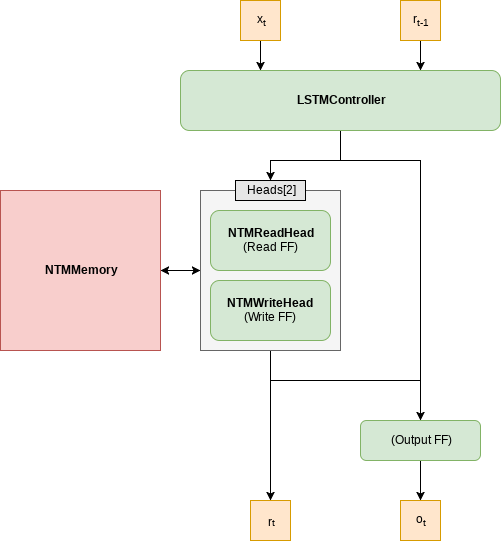### Features

• Batch learning support
• Numerically stable
• copy and repeat-copy experiments agree with the paper

The Copy task tests the NTM's ability to store and recall a long sequence of arbitrary information. The input to the network is a random sequence of bits, ending with a delimiter. The sequence lengths are randomised between 1 to 20.

### Training

Training convergence for the copy task using 4 different seeds (see the notebook for details)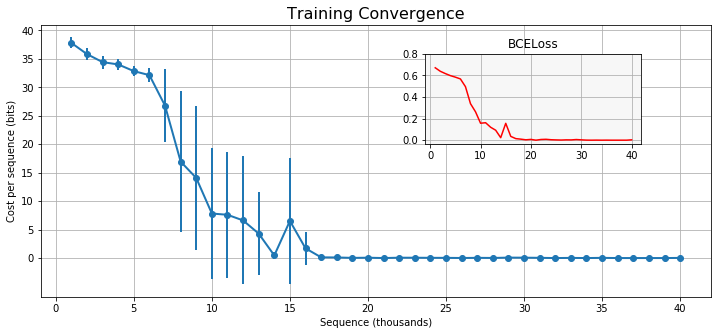The following plot shows the cost per sequence length during training. The network was trained with `seed=10` and shows fast convergence. Other seeds may not perform as well but should converge in less than 30K iterations.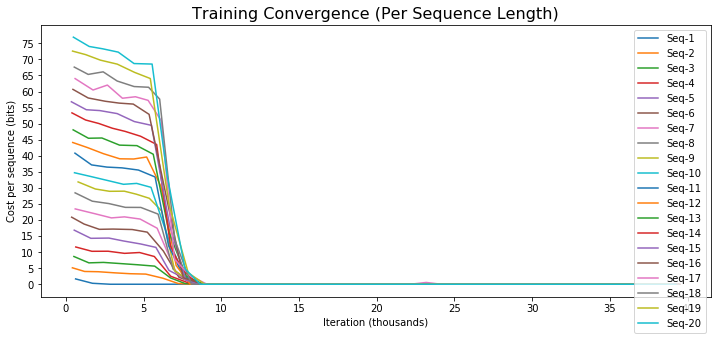### Evaluation

Here is an animated GIF that shows how the model generalize. The model was evaluated after every 500 training samples, using the target sequence shown in the upper part of the image. The bottom part shows the network output at any given training stage.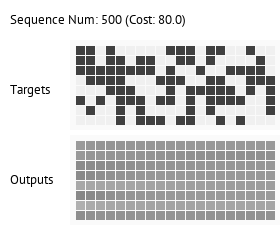The following is the same, but with `sequence length = 80`. Note that the network was trained with sequences of lengths 1 to 20.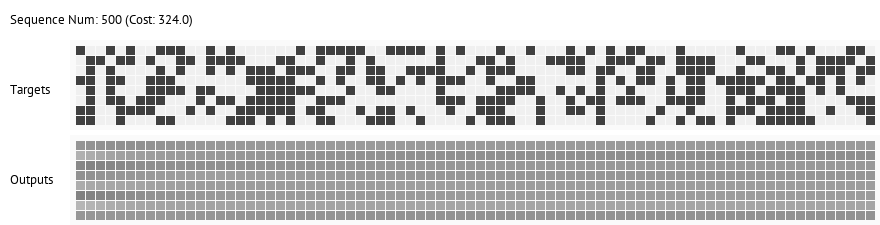The Repeat Copy task tests whether the NTM can learn a simple nested function, and invoke it by learning to execute a for loop. The input to the network is a random sequence of bits, followed by a delimiter and a scalar value that represents the number of repetitions to output. The number of repetitions, was normalized to have zero mean and variance of one (as in the paper). Both the length of the sequence and the number of repetitions are randomised between 1 to 10.

### Training

Training convergence for the repeat-copy task using 4 different seeds (see the notebook for details)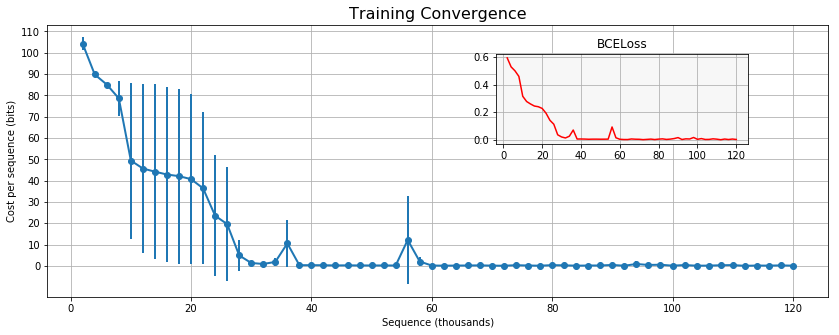### Evaluation

The following image shows the input presented to the network, a sequence of bits + delimiter + num-reps scalar. Specifically the sequence length here is eight and the number of repetitions is five.And here's the output the network had predicted: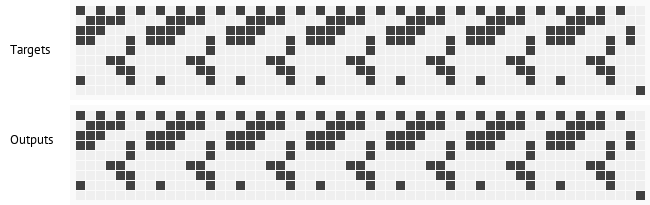Here's an animated GIF that shows how the network learns to predict the targets. Specifically, the network was evaluated in each checkpoint saved during training with the same input sequence.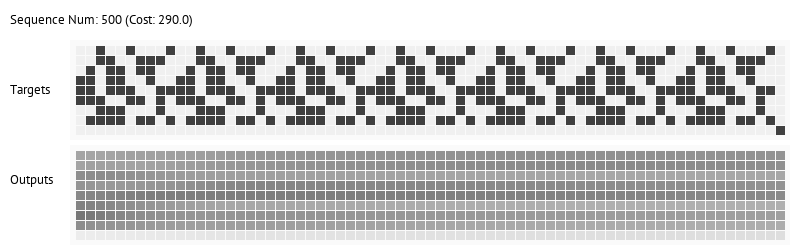## Installation

The NTM can be used as a reusable module, currently not packaged though.

1. Clone repository
2. Install PyTorch
3. pip install -r requirements.txt

## Usage

Execute ./train.py

``````usage: train.py [-h] [--seed SEED] [--task {copy,repeat-copy}] [-p PARAM]
[--checkpoint-interval CHECKPOINT_INTERVAL]
[--checkpoint-path CHECKPOINT_PATH]
[--report-interval REPORT_INTERVAL]

optional arguments:
-h, --help            show this help message and exit
--seed SEED           Seed value for RNGs
Choose the task to train (default: copy)
-p PARAM, --param PARAM
Override model params. Example: "-pbatch_size=4